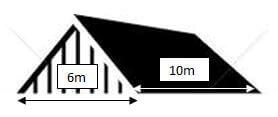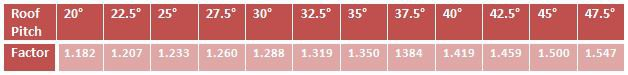Get Quote

# To work out what size of soakaway is required, you firstly need to calculate the area of the roof that will discharge into the soakaway. This is calculated as follows;

#### CALCULATING THE ROOF AREA

Measure the length of the roof from one end to the other along the eaves. Write this figure down on a notepad.

Then measure the width of the gable end, or the hip end, and divide this number by two. Write down on the notepad the figure that you get after dividing by 2.

Now multiply together the two figures on your pad. Here’s an example;The first figure you write down will be 10. The second figure will be 3 (6 divided by 2). Multiplying these two together gives 30 (10 x 3).

Now for the clever bit. To allow for the slope of the roof you need to multiply your roof area figure by the factor shown in the table below, depending upon the pitch of your roof.In our example above, let’s assume we have a roof pitch of 45°. Simply multiply our initial figure of 30 by 1.5 to give an effective roof area of 45m(30 x 1.5).

Note: If you are unsure of the exact pitch of your roof, choose a pitch that is more likely to be too steep than too shallow – most domestic roofs are pitched somewhere between 35° and 45°.

#### HOW MANY SOAKAWAY CRATES DO I NEED?

Once you have calculated your effective roof area, you can use the table below to work out how many Soakaway crates you may need.

 Effective Roof Area (Square Metres) Size of Soakaway crates needed (Cubic Metres) Number of Aquacrate Crates Required 25 m² 0.5m³ (500 litres) 1 30 m² 0.6m³ (600 litres) 2 40 m² 0.8m³ (800 litres) 3 50 m² 1.0m³ (1000 litres) 3 60 m² 1.2m³ (1200 litres) 4 80 m² 1.6m³ (1600 litres) 5 100 m² 2.0m³ (2000 litres) 6

Model: MEM100
Membrane 4.5m x 100m..
£236.80
Model: MEM5
Membrane 5m x 4.5m..
£19.20
Model: SCP1
Soakaway Crates 1m x 1m x 400mm 20T..
£62.08
Model: SCP2
Stormcrate 1m x 1m x 400 (2 Crates/Membrane/Tape)..
£138.24
Model: SCP3
Stormcrate 1m x 1m x 400 (3 Crates/Membrane/Tape)..
£197.12
Model: SCP4
Stormcrate 1m x 1m x 400 (4 Crates/Membrane/Tape)..
£258.56
Model: SCP6
Stormcrate 1m x 1m x 400 (6 Crates/Membrane/Tape)..
£390.40
Showing 1 to 7 of 7 (1 Pages)